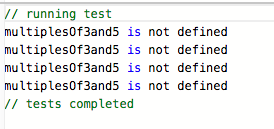# Problem 1: Multiples of 3 and 5 ---> tests not valid?

Tell us what’s happening:

It seems that tests for this challenge are not valid. I’ve tested my solution locally and results are as they should be.

``````
function multiplesOf3And5(number) {
const factors = [3, 5];
let resultsSet = new Set();
factors.forEach(factor => {
for (let i = 1; i < number; i++) {
let product = i * factor;
if (product < number) {
} else {
break;
}
};
});

const result = [...resultsSet].reduce((sum, num) => sum + num, 0);

return result;
}

multiplesOf3and5(1000);
``````

User Agent is: `Mozilla/5.0 (Windows NT 10.0; Win64; x64) AppleWebKit/537.36 (KHTML, like Gecko) Chrome/67.0.3396.99 Safari/537.36`.oh I can see it now… lol thanks